# Algebra ReviewPage 2

#### WATCH ALL SLIDES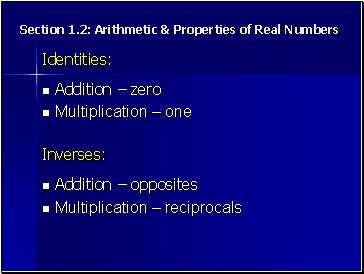Section 1.2: Arithetic & Properties of Real Numbers

Identities:

Multiplication – one

Inverses:

Multiplication – reciprocals

Slide 12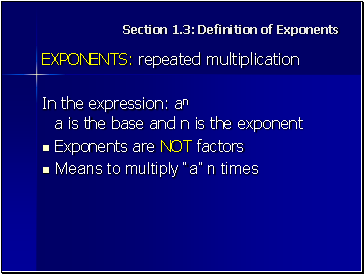Section 1.3: Definition of Exponents

## Exponents: repeated multiplication

In the expression: an a is the base and n is the exponent

Exponents are NOT factors

Means to multiply “a” n times

Slide 13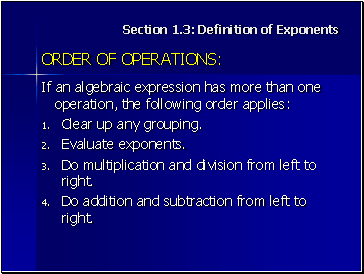Section 1.3: Definition of Exponents

## Order of operations

If an algebraic expression has more than one operation, the following order applies:

Clear up any grouping.

Evaluate exponents.

Do multiplication and division from left to right.

Do addition and subtraction from left to right.

Slide 14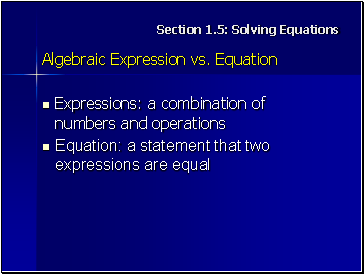Section 1.5: Solving Equations

## Algebraic Expression vs. Equation

Expressions: a combination of numbers and operations

Equation: a statement that two expressions are equal

Slide 15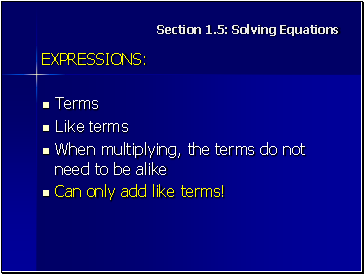Section 1.5: Solving Equations

## Expressions

Terms

Like terms

When multiplying, the terms do not need to be alike

Slide 16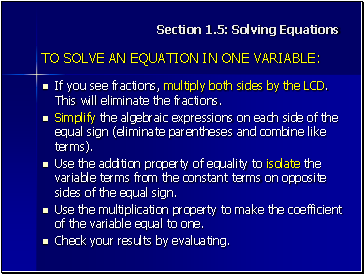Section 1.5: Solving Equations

## To solve an equation in one variable

If you see fractions, multiply both sides by the LCD. This will eliminate the fractions.

Simplify the algebraic expressions on each side of the equal sign (eliminate parentheses and combine like terms).

Use the addition property of equality to isolate the variable terms from the constant terms on opposite sides of the equal sign.

Use the multiplication property to make the coefficient of the variable equal to one.

Slide 17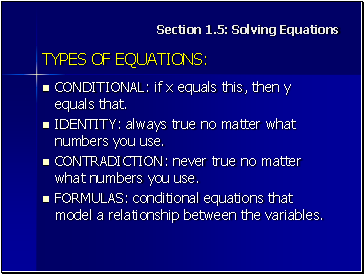Section 1.5: Solving Equations

## Types of equations

CONDITIONAL: if x equals this, then y equals that.

IDENTITY: always true no matter what numbers you use.

CONTRADICTION: never true no matter what numbers you use.

FORMULAS: conditional equations that model a relationship between the variables.

Slide 18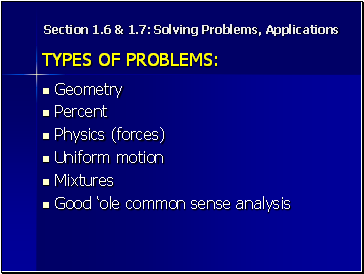Section 1.6 & 1.7: Solving Problems, Applications

## Types of problems

Geometry

Percent

Physics (forces)

Uniform motion

Mixtures

Good ‘ole common sense analysis

Slide 19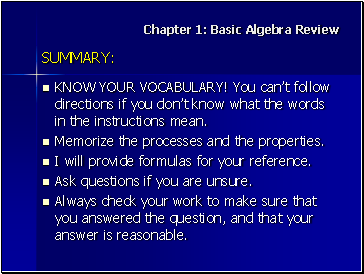Go to page:
1  2  3